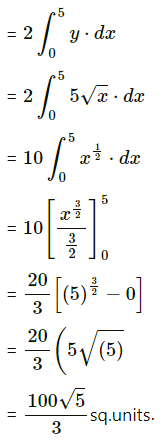Balbharati Maharashtra State Board Std 12 Commerce Statistics Part 1 Digest Pdf Chapter 7 Application of Definite Integration Miscellaneous Exercise 7 Questions and Answers.

## Maharashtra State Board 12th Commerce Maths Solutions Chapter 7 Application of Definite Integration Miscellaneous Exercise 7

(I) Choose the correct alternatives:

Question 1.
Area of the region bounded by the curve x2 = y, the X-axis and the lines x = 1 and x = 3 is
(a) $$\frac{26}{3}$$ sq units
(b) $$\frac{3}{26}$$ sq units
(c) 26 sq units
(d) 3 sq units
(a) $$\frac{26}{3}$$ sq units

Question 2.
The area of the region bounded by y2 = 4x, the X-axis and the lines x = 1 and x = 4 is
(a) 28 sq units
(b) 3 sq unit
(c) $$\frac{28}{3}$$ sq units
(d) $$\frac{3}{28}$$ sq units
(c) $$\frac{28}{3}$$ sq unitsQuestion 3.
Area of the region bounded by x2 = 16y, y = 1 and y = 4 and the Y-axis, lying in the first quadrant is
(a) 63 sq units
(b) $$\frac{3}{56}$$ sq units
(c) $$\frac{56}{3}$$ sq units
(d) $$\frac{63}{7}$$ sq units
(c) $$\frac{56}{3}$$ sq units

Question 4.
Area of the region bounded by y = x4, x = 1, x = 5 and the X-axis is
(a) $$\frac{3142}{5}$$ sq units
(b) $$\frac{3124}{5}$$ sq units
(c) $$\frac{3142}{3}$$ sq units
(d) $$\frac{3124}{3}$$ sq units
(b) $$\frac{3124}{5}$$ sq units

Question 5.
Using definite integration area of circle x2 + y2 = 25 is
(a) 5π sq units
(b) 4π sq units
(c) 25π sq units
(d) 25 sq units
(c) 25π sq units

(II) Fill in the blanks:

Question 1.
Area of the region bounded by y = x4, x = 1, x = 5 and the X-axis is _________
$$\frac{3124}{5}$$ sq units

Question 2.
Using definite integration area of the circle x2 + y2 = 49 is ___________
49π sq units

Question 3.
Area of the region bounded by x2 = 16y, y = 1, y = 4 and the Y-axis lying in the first quadrant is _________
$$\frac{56}{3}$$ sq unitsQuestion 4.
The area of the region bounded by the curve x2 = y, the X-axis and the lines x = 3 and x = 9 is _________
234 sq units

Question 5.
The area of the region bounded by y2 = 4x, the X-axis and the lines x = 1 and x = 4 is __________
$$\frac{28}{3}$$ sq units

(III) State whether each of the following is True or False.

Question 1.
The area bounded by the curve x = g(y), Y-axis and bounded between the lines y = c and y = d is given by $$\int_{c}^{d} x d y=\int_{y=c}^{y=d} g(y) d y$$
True

Question 2.
The area bounded by two curves y = f(x), y = g(x) and X-axis is $$\left|\int_{a}^{b} f(x) d x-\int_{b}^{a} g(x) d x\right|$$
False

Question 3.
The area bounded by the curve y = f(x), X-axis and lines x = a and x = b is $$\left|\int_{a}^{b} f(x) d x\right|$$
TrueQuestion 4.
If the curve, under consideration, is below the X-axis, then the area bounded by curve, X-axis, and lines x = a, x = b is positive.
False

Question 5.
The area of the portion lying above the X-axis is positive.
True

(IV) Solve the following:

Question 1.
Find the area of the region bounded by the curve xy = c2, the X-axis, and the lines x = c, x = 2c.
Solution: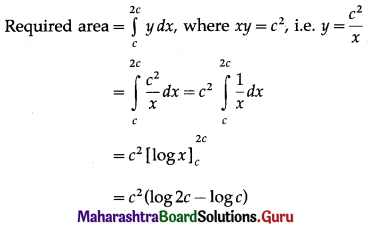= c2 log($$\frac{2 c}{c}$$)
= c2 . log 2 sq units.

Question 2.
Find the area between the parabolas y2 = 7x and x2 = 7y.
Solution: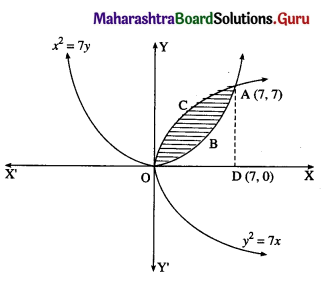For finding the points of intersection of the two parabolas,
we equate the values of y2 from their equations.
From the equation x2 = 7y, y2 = $$\frac{x^{4}}{49}$$
∴ $$\frac{x^{4}}{49}$$ = 7x
∴ x4 = 343x
∴ x4 – 343x = 0
∴ x(x3 – 343) = 0
∴ x = 0 or x3 = 343, i.e. x = 7
When x = 0, y = 0
When x = 7, 7y = 49
∴ y = 7
∴ the points of intersection are O(0, 0) and A(7, 7)
Required area = area of the region OBACO
= (area of the region ODACO) – (area of the region ODABO)
Now, area of the region ODACO = area under the parabola y2 = 7x
i.e. y = √7 √x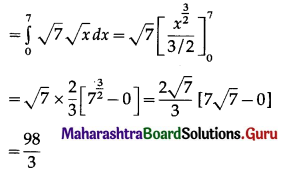Area of the region ODABO = Area under the parabola
x2 = 7y
i.e. y = $$\frac{x^{2}}{7}$$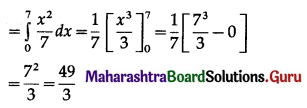∴ required area = $$\frac{98}{3}-\frac{49}{3}=\frac{49}{3}$$ sq units.Question 3.
Find the area of the region bounded by the curve y = x2 and the line y = 10.
Solution: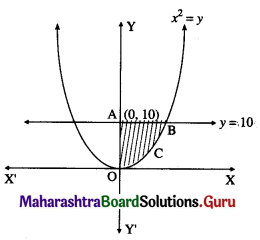By the symmetry of the parabola,
the required area is twice the area of the region OABCO
Now, the area of the region OABCO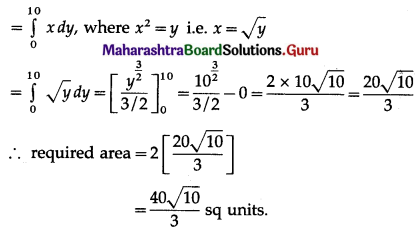Question 4.
Find the area of the ellipse $$\frac{x^{2}}{16}+\frac{y^{2}}{9}$$ = 1.
Solution:
By the symmetry of the ellipse, the required area of the ellipse is 4 times the area of the region OPQO.
For the region OPQO, the limits of integration are x = 0 and x = 4.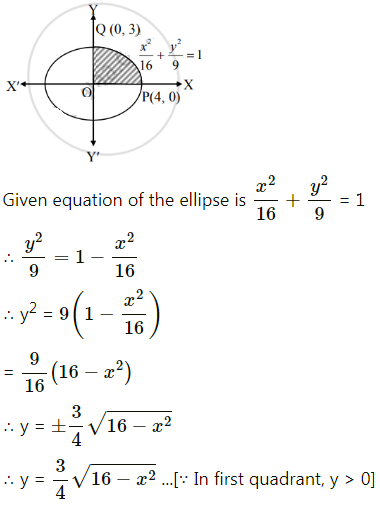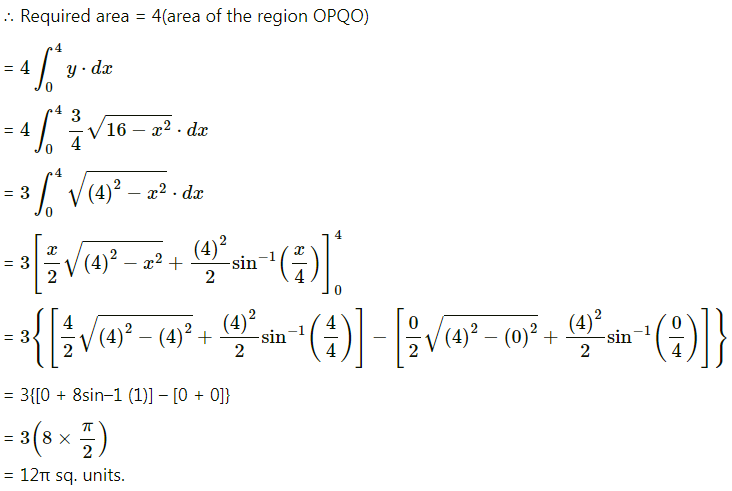Question 5.
Find the area of the region bounded by y = x2, the X-axis and x = 1, x = 4.
Solution:
Required area = $$\int_{1}^{4} y d x$$, where y = x2
= $$\int_{1}^{4} x^{2} d x$$
= $$\left[\frac{x^{3}}{3}\right]_{1}^{4}=\frac{4^{3}}{3}-\frac{1}{3}=\frac{64-1}{3}$$
= 21 sq units.

Question 6.
Find the area of the region bounded by the curve x2 = 25y, y = 1, y = 4, and the Y-axis.
Solution: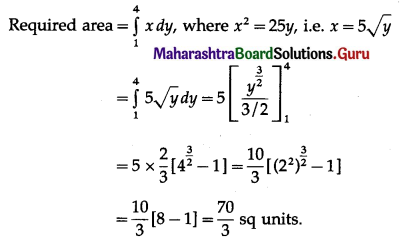Question 7.
Find the area of the region bounded by the parabola y2 = 25x and the line x = 5.
Solution: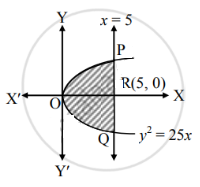Given the equation of the parabola is y2 = 25x
∴ y = 5√x …… [∵ IIn first quadrant, y > 0]
Required area = area of the region OQRPO
= 2(area of the region ORPO)